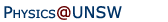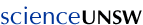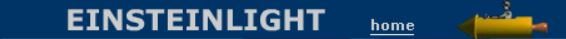Relativity in brief... or in detail..

## The Lorentz transform equations, the addition of velocities and spacetime

• Galilean transform equations,
• Lortentz transformation equations
• Addition of velocities in special relativity

• Spacetime

#### Galilean transform equations

In An introduction to the mechanics of Galileo and Newton, we saw that converting between two inertial frames was easy in that version of mechanics. Let's consider motion in the x direction, with relative speed v: the train goes past the station at v (on a straight and level track that we call the x axis). We synchronise watches (set t = 0) at some convenient time, let's say when the back of the train passes Jasper, the observer in the station. So the position of the back of the train is x = vt, where t is the time elapsed. If Zoe, a passenger in the train, is a distance x' ahead of the back of the train, then Jasper sees her position as x = vt + x', where we use the prime (') to signify Zoe's frame and the absence of it to signify Jasper's.

Formally, we should consider the y and z directions too. In Galilean relativity, there is no reason for Jasper and Zoe to disagree on how far she is above the floor (so z = z') or how far sideways she is from the aisle (so y = y'). Further, we've made the important assumption (for Galilean relativity) that their measurements of time are the same, so Zoe's time t' = t. So the transform equations for Galilean relativity (motion v in the x direction) are:

x = vt + x',
y = y',
z = z', and
t = t'.
We've already seen that, if Zoe walks at speed u' and acceleration a', Jasper sees her speed u with respect to him as:
u = v + u', and
a = a'
for motion in the x direction. Now we can see that this follows from the equations above by differentiation (twice).

For relativity, we need new transform equations because, as we have seen in Module 3, when v becomes comparable with the speed of light, Zoe and Jasper disagree on time and length.

#### Lorentz transform equations

So, let's look for new transformation equations relating (x,y,z,t) and (x',y',z,t'). The motion is only in the x direction. They can again synchronise clocks: for convenience and symmetry, when they are side by side, they call that position zero and time zero. So x = 0 at t = 0 and x' = 0 at t' = 0. Further, at this moment, they can check that measurements in the y and z directions coincide, so two of the transformation equations are easy: y = y' and z = z'. Same as Galileo.

However, we know that they disagree over time and length, so we need new transform equations for x and t. There is a good reason to try equations that are linear in x and t. First, we've seen that the time dilation and length contraction are linear in time and length. Second, if they are not linear, then we could end up have two solutions of x and t for a single event. This would be awkward for a frame that's travelling with constant velocity v! So let's write:

x' = Ax + Bt,
y' = y,
z' = z,
t' = Ct + Dx.
where A, B, C and D are constants (i.e. independent of t and x: they will, however, be functions of v.) At v = 0, we can see that A and C = 1, while B and D = 0. Now x' = 0 marks the back of the train, so x = vt. Substituting in the first equation gives
A = −B/v.
At t = 0, Jasper sees Zoe's length contraction, so
x' = γx = Ax
and using these equations we get:
x' = γ(x - vt).
Now let's look at time dilation, again from Jasper's point of view. At x = 0, he sees Zoe's clock running slow, so t'  = γt, which gives
C = γ.
Now let's look from the point of view of the back of the train, where x' = 0. From this position, Zoe sees Jasper's clock running slow, so at x' = 0, we have t = γt'. We also know that the train is moving at v with respect to Jasper, so for the position x' = 0, we also have x =  vt. Substituting these into the last transformation equation gives
t/γ = γt - Dvt,     whence
1 = γ2 - Dγv      and so
D = (γ2 - 1)/γv.
This gives us the Lorentz transformation equations:We should, of course, check that we get the correct length contraction and time dilation. This can be done by considering the particular cases of t = 0 and x = 0. And of course we should check that, in the limit where v is very much less than c, that these equations reduce to the Galilean transform above. If we set γ approximately equal to one, and v/c very much less than one, these equations do indeed reduce to the ones we use for Galilean relativity.

#### Addition of velocities in special relativity

What about relative velocities? Now that we have the transformation equations, that is just a matter of taking derivatives. Let ux' be the x component of Zoe's velocity, measured in the train, and we have: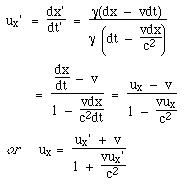so the rule for adding velocities is more difficult in Einstein's relativity than in Galileo's. Let's now do a couple of examples.

Imagine that a Vogon star ship travels towards Earth at v = c/2. The Vogon captain, with a poetic flourish, fires a sonnet (the name of a particularly dangerous Vogon armament) at Earth with speed of c/2, with respect to the star ship. With what relative speed does the sonnet travel towards Earth? Well this is just a case of substitution in the above equation, which yields: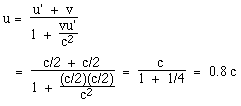So it came out less than c — less than it would have under Galileo or Newton's rules. Yes, we warned you that it was weird, but once again remind you that your common sense (the standard against which we judge weirdness) was developed and tested on very slow moving objects. (See The weirdness — and the logic — of the principle of invariance.) If we take the two speeds u' and v both as 300 kilometres per second (u' = 0.0010*c and v = 0.0010*c) and substitute in these equations, we get u = 0.0020*c = 600 km/s. So the relativistic equations are consistent with our common sense, even at 300 kilometres per second, which is much faster than everyday objects (1000 times faster than an airliner).

Okay, now cut to the chase. In one of the animations, Zoe (travelling at c/2) turns her headlights on, and both she and Jasper record their speed as c. Does that come out of these equations?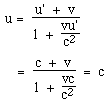So yes, it does. Which is just as well. The whole starting point was that electromagnetism was the same for both observers, so both must see light travel at c. Weird, but true.

One further important observation. The equation for calculating relative velocities is non linear in ux', unless ux' is very much less than c. One important consequence of this is that, using the definition of momentum p = mu, we would find that momentum is not conserved. In relativity, we must use p = γmu so that p is conserved in the absence of external forces. An easy way to check this is to consider a symmetric inelastic collision in the two frames. We return to this in Energy in Newtonian mechanics and in relativity.

#### Spacetime

So, under these transform equations, can our observers agree on anything? Well yes, there is one thing. Let's take an event (conventionally a spark, so it is well localised in time and space and radiates light in all directions) at (x,0,0,t) and another at (x1,0,0,t1). The quantity defined by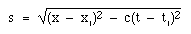is the same for all observers, as you can check by substitution. (The substitution is less messy if you set (x1,t1) = (x'1,t'1) = (0,0), which you can do with no loss of generality by suitable choice of origin and synchronisation.) So, we could generalise this for events (x,y,z,t) and (x1,y1,z1,t1) that differed in y and z position, too:Note the asymmetry concerning time. First, it has different units. This can be regarded as an artifact of our system of units, and can be solved by measuring the time as ct instead of c. However, in the equations above, the time term has a minus sign, whereas the spatial dimensions all have plus signs. We can remove this asymmetry by taking the minus sign inside the square root, and using the imaginary (in the mathematical sense) number i where i2 = -1. We can now writewhere (x,y,z,ict) are the coordinates in spacetime, a four dimensional continuum in which all observers in uniform motion agree on 'lengths' expressed by s.

You will have noticed that time is different from the three spatial dimensions. To some extent, this 'specialness' of time appears in the fact that is an imaginary coordinate, and that it has the factor c in it. (Another way of saying the latter is that its natural units are inconveniently sized, or that human units of time and length, m/s, are suitable for speeds much slower than the natural speed in electromagnetism. i.e. a light second is much greater than a metre).

But there is more. You can walk North or South with equal ease, and East and West. Travelling up and down are also possible. Time travel, on the other hand is easy in the forwards direction (we are all doing it) but not in the reverse. This takes us away from the physics that appears in this volume (electromagnetism, relativity, mechanics and quantum mechanics) and into thermal physics and the quantity entropy, whose symbol is S. S is related to heat and temperature, which in turn are related to energies distributed on the molecular scale. If you can arrange to do a thermal process in a way that is reversible, then the entropy of a system increases by ΔS where ΔS is the ratio ΔQ/T, i.e. the heat you add (ΔQ) divided by the temperature T at which you add it.

The second law of thermodynamics can be stated in several ways. The way I like to state it is this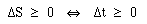where the symbol in the middle means "implies and is implied by". I think that the symmetry is important. What we call forward travel in time is the direction in which entropy doesn't decrease, which is the direction in which chemicals burn and therefore memories are made and things (including people) age. And of course you'd want ask why, and what I mean by the rather generalised sense of 'burn'. Then there is the question of whether the thermodynamic arrow of time is necessarily the same as the cosmological one, the one in which the universe expands and cools. But this has taken us far from the topic and would be the subject of a whole further (interesting) presentation. For another time.

•Home | Summary | Quiz | Credits School of Physics - The University of New South Wales Sydney, Australia 2052 © School of Physics UNSW 2052 Disclaimer feedback